### Home > CAAC > Chapter 13 > Lesson 13.CS1-S > Problem4-135

4-135.
1. For each quadratic function below, use the idea of completing the square to write it in graphing form. Then state the vertex of each parabola. Homework Help ✎

1. f(x) = x2 + 6x + 15

2. y = x2 − 4x + 9

3. f(x) = x2 + 8x

4. y = x2 + 5x − 2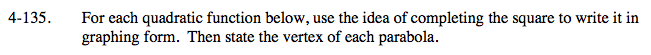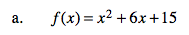How many ones do you need to make a perfect square? How many do you have?

f(x) = (x + 3)2 +6
vertex: (−3, 6)
axis of symmetry: x = − 3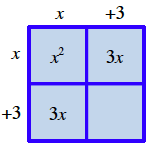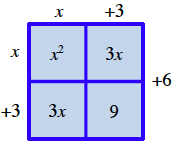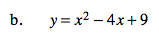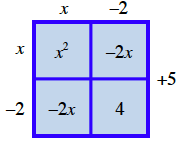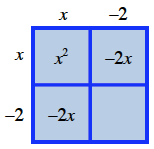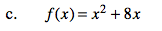Write each of the expressions in its proper location.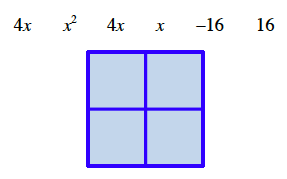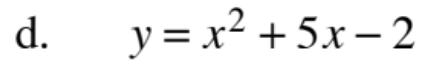y = (x + 2.5)2 − 8.25